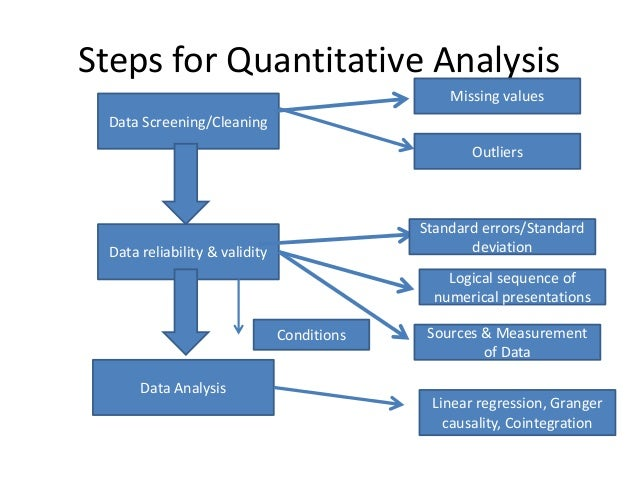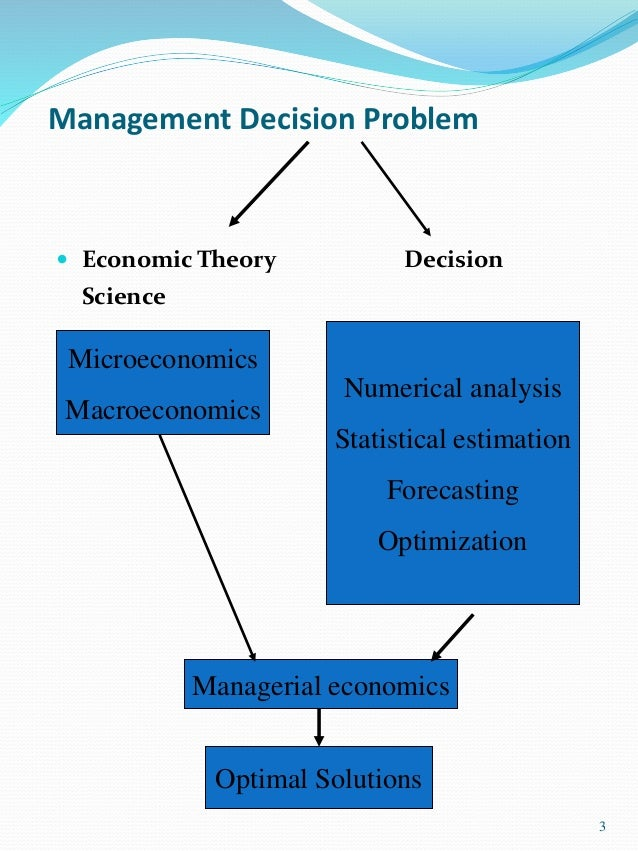It looks like you're using Internet Explorer 11 or older. This website works best with modern browsers such as the latest versions of Chrome, Firefox, Safari, and Edge. If you continue with this browser, you may see unexpected results.

# Numerical Analysis: Home

Objective: Teach the use of computers for the numerical solution of engineering problems

## Introduction

Numerical analysis is the study of algorithm that use numerical approximation (as opposed to general symbolic manipulations) for the problems of mathematical analysis (as distinguished from discrete mathematics).

One of the earliest mathematical writings is a Babylonian tablet from the Yale Babylonian Collection (YBC 7289), which gives a sexagesimal numerical approximation of, the length of the diagonal  in a unit square. Being able to compute the sides of a triangle  is extremely important, for instance, in astronomy, carpentry and construction.

Numerical analysis continues this long tradition of practical mathematical calculations. Much like the Babylonian approximation of, modern numerical analysis does not seek exact answers, because exact answers are often impossible to obtain in practice. Instead, much of numerical analysis is concerned with obtaining approximate solutions while maintaining reasonable bounds on errors.

## Level## Related Images